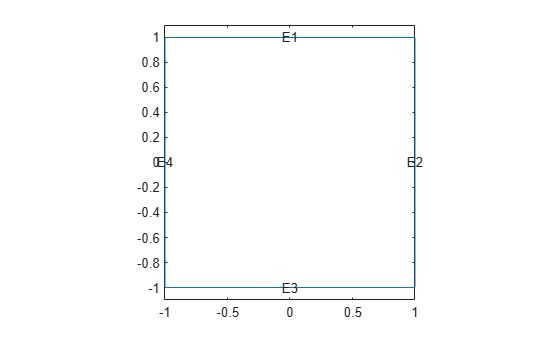# findThermalBC

Find thermal boundary conditions assigned to a geometric region

## Syntax

``tbca = findThermalBC(thermalmodel.BoundaryConditions,RegionType,RegionID)``

## Description

example

````tbca = findThermalBC(thermalmodel.BoundaryConditions,RegionType,RegionID)` returns the thermal boundary condition assigned to the specified region.```

## Examples

collapse all

Create a thermal model and include a square geometry.

```thermalmodel = createpde('thermal'); geometryFromEdges(thermalmodel,@squareg); pdegplot(thermalmodel,'EdgeLabels','on') ylim([-1.1 1.1]) axis equal```Apply temperature boundary conditions on edges 1 and 3 of the square.

`thermalBC(thermalmodel,'Edge',[1 3],'Temperature',100);`

Apply a heat flux boundary condition on edge 4 of the square.

`thermalBC(thermalmodel,'Edge',4,'HeatFlux',20);`

Check the boundary condition specification on edge 1.

`tbcaEdge1 = findThermalBC(thermalmodel.BoundaryConditions,'Edge',1)`
```tbcaEdge1 = ThermalBC with properties: RegionType: 'Edge' RegionID: [1 3] Temperature: 100 HeatFlux: [] ConvectionCoefficient: [] Emissivity: [] AmbientTemperature: [] Vectorized: 'off' Label: [] ```

Check the boundary condition specifications on edges 3 and 4.

```tbca = findThermalBC(thermalmodel.BoundaryConditions,'Edge',[3:4]); tbcaEdge3 = tbca(1)```
```tbcaEdge3 = ThermalBC with properties: RegionType: 'Edge' RegionID: [1 3] Temperature: 100 HeatFlux: [] ConvectionCoefficient: [] Emissivity: [] AmbientTemperature: [] Vectorized: 'off' Label: [] ```
`tbcaEdge4 = tbca(2)`
```tbcaEdge4 = ThermalBC with properties: RegionType: 'Edge' RegionID: 4 Temperature: [] HeatFlux: 20 ConvectionCoefficient: [] Emissivity: [] AmbientTemperature: [] Vectorized: 'off' Label: [] ```

Create a thermal model and include a block geometry.

```thermalmodel = createpde('thermal','transient'); gm = importGeometry(thermalmodel,'Block.stl'); pdegplot(thermalmodel,'FaceLabels','on','FaceAlpha',0.5)```Apply temperature boundary condition on faces 1 and 3 of a block.

```thermalBC(thermalmodel,'Face',1,'Temperature',100); thermalBC(thermalmodel,'Face',3,'Temperature',300);```

Apply convection boundary condition on faces 5 and 6 of a block.

```thermalBC(thermalmodel,'Face',[5,6],... 'ConvectionCoefficient',5,... 'AmbientTemperature',27);```

Check the boundary condition specification on faces 1 and 3.

```tbca = findThermalBC(thermalmodel.BoundaryConditions,'Face',[1,3]); tbcaFace1 = tbca(1)```
```tbcaFace1 = ThermalBC with properties: RegionType: 'Face' RegionID: 1 Temperature: 100 HeatFlux: [] ConvectionCoefficient: [] Emissivity: [] AmbientTemperature: [] Vectorized: 'off' Label: [] ```
`tbcaFace3 = tbca(2)`
```tbcaFace3 = ThermalBC with properties: RegionType: 'Face' RegionID: 3 Temperature: 300 HeatFlux: [] ConvectionCoefficient: [] Emissivity: [] AmbientTemperature: [] Vectorized: 'off' Label: [] ```

Check the boundary condition specifications on faces 5 and 6.

`tbcaFace5 = findThermalBC(thermalmodel.BoundaryConditions,'Face',5)`
```tbcaFace5 = ThermalBC with properties: RegionType: 'Face' RegionID: [5 6] Temperature: [] HeatFlux: [] ConvectionCoefficient: 5 Emissivity: [] AmbientTemperature: 27 Vectorized: 'off' Label: [] ```
`tbcaFace6 = findThermalBC(thermalmodel.BoundaryConditions,'Face',6)`
```tbcaFace6 = ThermalBC with properties: RegionType: 'Face' RegionID: [5 6] Temperature: [] HeatFlux: [] ConvectionCoefficient: 5 Emissivity: [] AmbientTemperature: 27 Vectorized: 'off' Label: [] ```

## Input Arguments

collapse all

Boundary conditions of a thermal model, specified as the `BoundaryConditions` property of a `ThermalModel` object.

Example: `thermalmodel.BoundaryConditions`

Geometric region type, specified as `'Face'` for 3-D geometry or `'Edge'` for 2-D geometry.

Data Types: `char` | `string`

Geometric region ID, specified as a vector of positive integers. Find the region IDs using `pdegplot` with the `'FaceLabels'` (3-D) or `'EdgeLabels'` (2-D) value set to `'on'`.

Data Types: `double`

## Output Arguments

collapse all

Thermal boundary condition for a particular region, returned as a `ThermalBC` object.